Next: The Harmonic Oscillator Up: Some Analytically Soluble Problems Previous: Some Analytically Soluble Problems

## The Particle in a Box

Consider a particle constrained to move in a single dimension, under the influence of a potential V(x) which is zero for
and infinite elsewhere. Since the wavefunction is not allowed to become infinite, it must have a value of zero where V(x) is infinite, so
is nonzero only within [0,a]. The Schrödinger equation is thus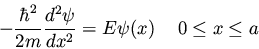(115)

It is easy to show that the eigenvectors and eigenvalues of this problem are
(116)
(117)

Extending the problem to three dimensions is rather straightforward; see McQuarrie , section 6.1.